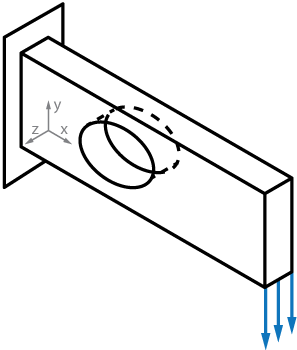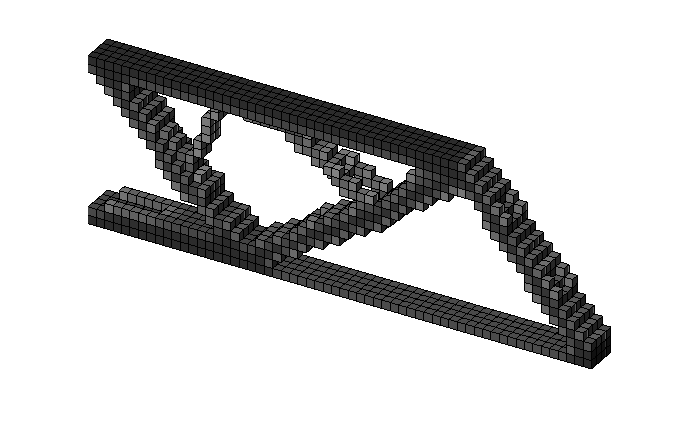# Active and passive elements - Top3d

In some designs, some elements may be desired to be solid or void (active or passive). In this tutorial you will learn how to set active and passive elements in the topology optimization program.

In some designs, some elements may be desired to be solid or void (active or passive). A $\texttt{nely} \times \texttt{nelx}\times \texttt{nelz}$ matrix with ones at elements desired to be solid, and a $\texttt{nely}\times \texttt{nelx}\times \texttt{nelz}$ matrix with zeros at elements desired to be void can be added to the program.

To solve the problem as shown below, the passive elements need to be defined first.# Step.1: Define active/passive elements

The passive elements are defined by adding the following lines after line 62:

for ely = 1:nely
for elx = 1:nelx
if sqrt((ely-nely/2.)^2+(elx-nelx/3.)^2) < nely/3.
passive(ely,elx) = 1;
else
passive(ely,elx) = 0;
end
end
end
passive = repmat(passive,[1,1,nelz]);
x(find(passive)) = 0;


# Step.2: Modify OC subroutine

One line is added in the OC subroutine under line 85:

xnew(find(passive)) = 0;

# Step.3: Run the program

The optimized beam shown belown is promoted by the line

top3d(60,20,4,0.3,3.0,1.5)